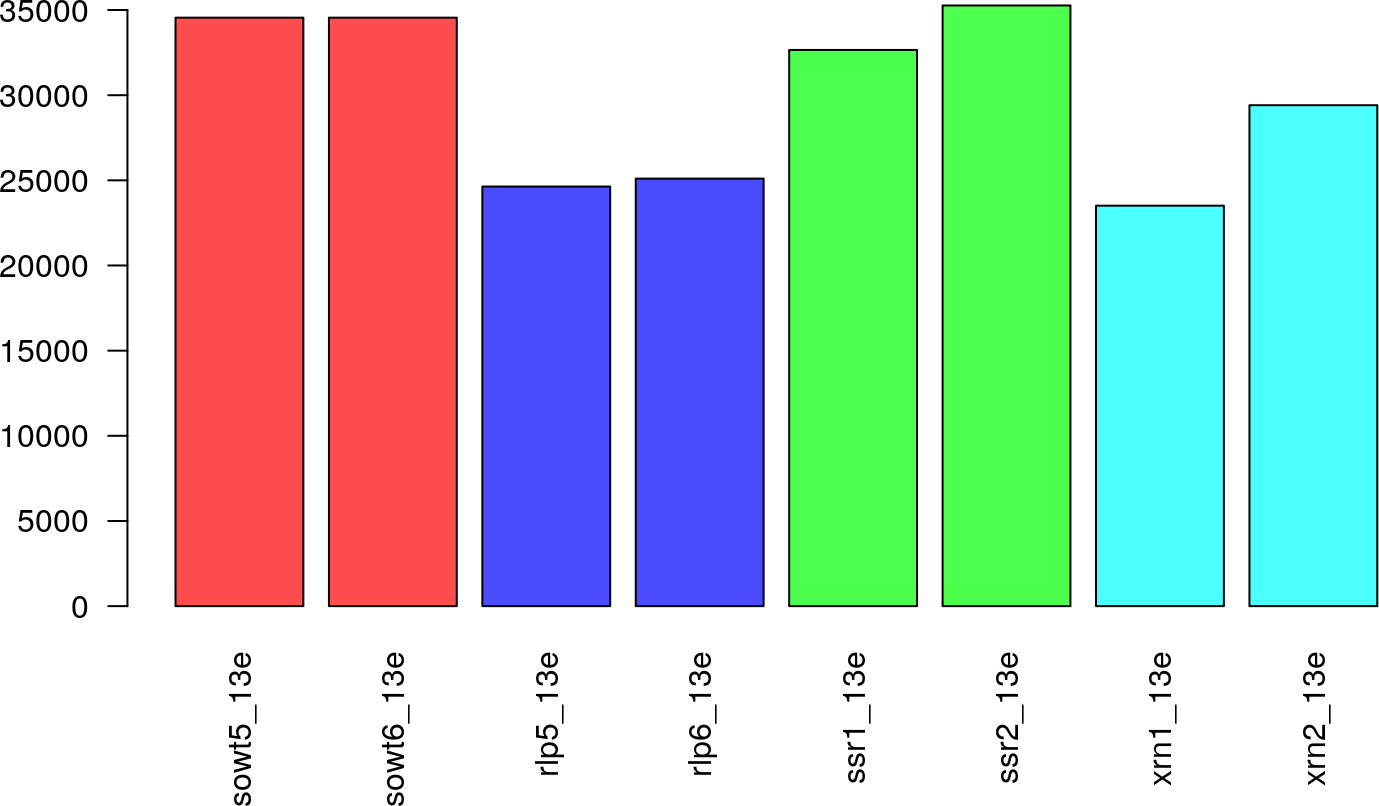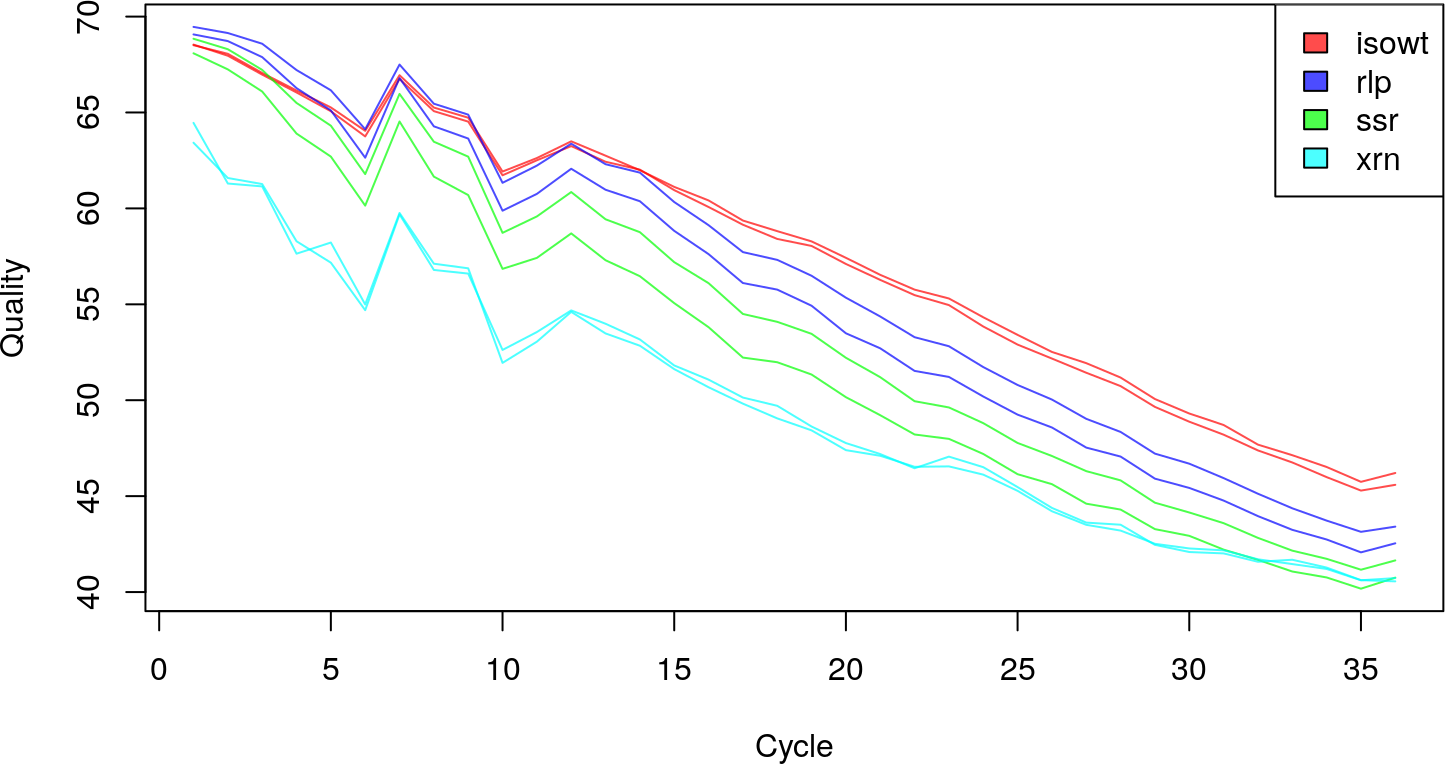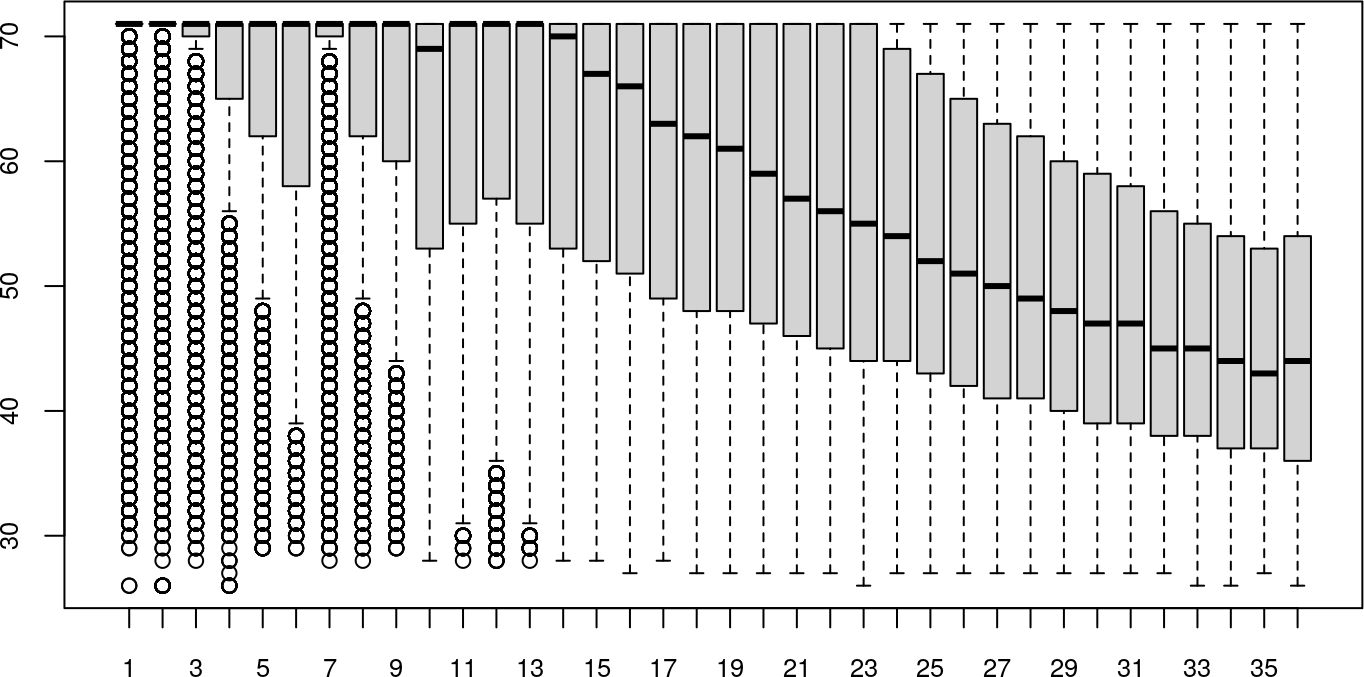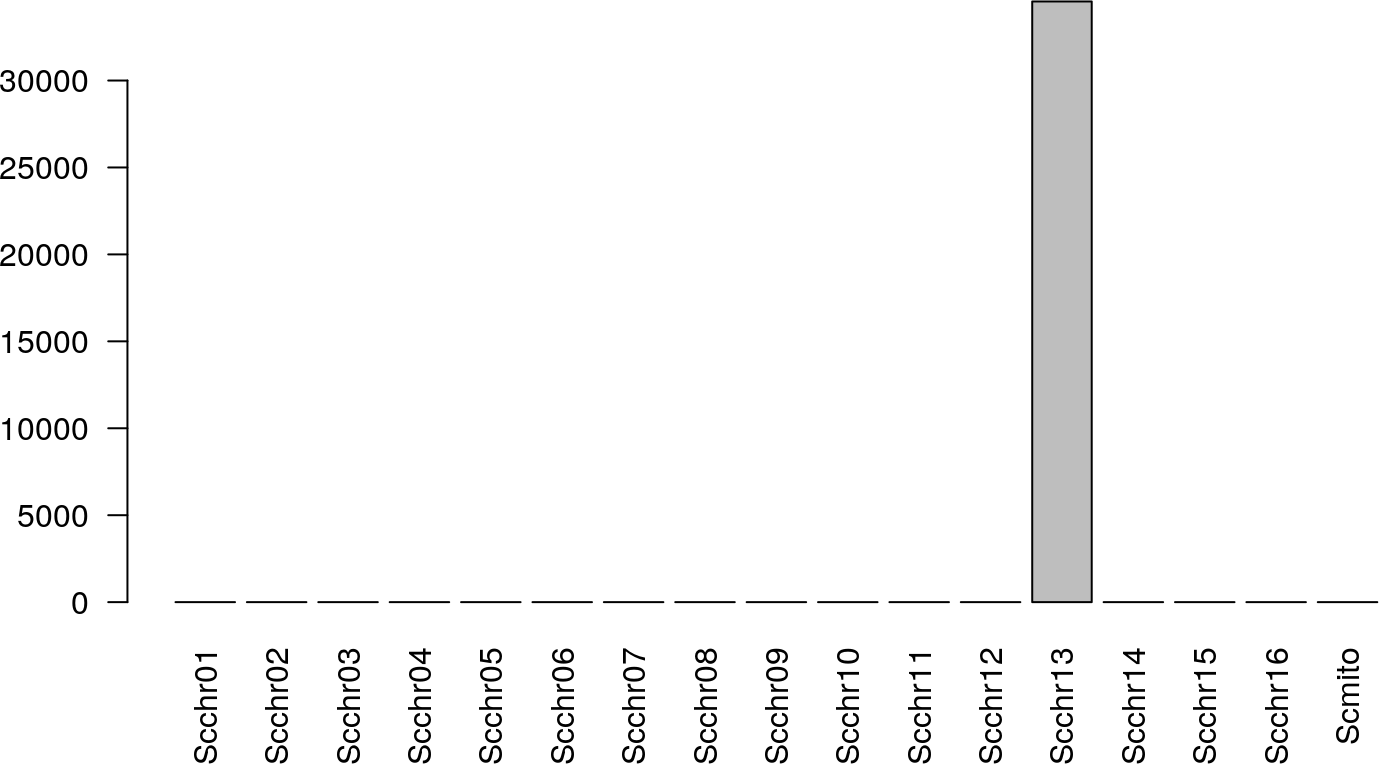# 1 Introduction

In this document, we show how to conduct Exploratory Data Analysis (EDA) and normalization for a typical RNA-Seq experiment using the package `EDASeq`.

One can think of EDA for RNA-Seq as a two-step process: “read-level” EDA helps in discovering lanes with low sequencing depths, quality issues, and unusual nucleotide frequencies, while ``gene-level’’ EDA can capture mislabeled lanes, issues with distributional assumptions (e.g., over-dispersion), and GC-content bias.

The package also implements both “within-lane” and “between-lane” normalization procedures, to account, respectively, for within-lane gene-specific (and possibly lane-specific) effects on read counts (e.g., related to gene length or GC-content) and for between-lane distributional differences in read counts (e.g., sequencing depths).

To illustrate the functionality of the `EDASeq` package, we make use of the Saccharomyces cerevisiae RNA-Seq data from (Lee et al. 2008). Briefly, a wild-type strain and three mutant strains were sequenced using the Solexa 1G Genome Analyzer. For each strain, there are four technical replicate lanes from the same library preparation. The reads were aligned using `Bowtie` (Langmead et al. 2009), with unique mapping and allowing up to two mismatches.

The `leeBamViews` package provides a subset of the aligned reads in BAM format. In particular, only the reads mapped between bases 800,000 and 900,000 of chromosome XIII are considered. We use these reads to illustrate read-level EDA.

The `yeastRNASeq` package contains gene-level read counts for four lanes: two replicates of the wild-type strain (“wt”) and two replicates of one of the mutant strains (“mut”). We use these data to illustrate gene-level EDA.

``````library(EDASeq)
library(yeastRNASeq)
library(leeBamViews)``````

Unaligned (unmapped) reads stored in FASTQ format may be managed via the class `FastqFileList` imported from `ShortRead`. Information related to the libraries sequenced in each lane can be stored in the `elementMetadata` slot of the `FastqFileList` object.

``````files <- list.files(file.path(system.file(package = "yeastRNASeq"),
"reads"), pattern = "fastq", full.names = TRUE)
names(files) <- gsub("\\.fastq.*", "", basename(files))
met <- DataFrame(conditions=c(rep("mut",2), rep("wt",2)),
row.names=names(files))
fastq <- FastqFileList(files)
fastq``````
``````## FastqFileList of length 4
## names(4): mut_1_f mut_2_f wt_1_f wt_2_f``````

The package can deal with aligned (mapped) reads in BAM format, using the class `BamFileList` from `Rsamtools`. Again, the `elementMetadata` slot can be used to store lane-level sample information.

``````files <- list.files(file.path(system.file(package = "leeBamViews"), "bam"),
pattern = "bam\$", full.names = TRUE)
names(files) <- gsub("\\.bam", "", basename(files))

gt <- gsub(".*/", "", files)
gt <- gsub("_.*", "", gt)
lane <- gsub(".*(.)\$", "\\1", gt)
geno <- gsub(".\$", "", gt)

pd <- DataFrame(geno=geno, lane=lane,
row.names=paste(geno,lane,sep="."))

bfs <- BamFileList(files)
bfs``````
``````## BamFileList of length 8
## names(8): isowt5_13e isowt6_13e ... xrn1_13e xrn2_13e``````

## 3.1 Numbers of unaligned and aligned reads

One important check for quality control is to look at the total number of reads produced in each lane, the number and the percentage of reads mapped to a reference genome. A low total number of reads might be a symptom of low quality of the input RNA, while a low mapping percentage might indicate poor quality of the reads (low complexity), problems with the reference genome, or mislabeled lanes.

``````colors <- c(rep(rgb(1,0,0,alpha=0.7),2),
rep(rgb(0,0,1,alpha=0.7),2),
rep(rgb(0,1,0,alpha=0.7),2),
rep(rgb(0,1,1,alpha=0.7),2))
barplot(bfs,las=2,col=colors)``````The figure, produced using the `barplot` method for the `BamFileList` class, displays the number of mapped reads for the subset of the yeast dataset included in the package `leeBamViews`. Unfortunately, `leeBamViews` does not provide unaligned reads, but barplots of the total number of reads can be obtained using the `barplot` method for the `FastqFileList` class. Analogously, one can plot the percentage of mapped reads with the `plot` method with signature `c(x="BamFileList", y="FastqFileList")`. See the manual pages for details.

As an additional quality check, one can plot the mean per-base (i.e., per-cycle) quality of the unmapped or mapped reads in every lane.

``````plotQuality(bfs,col=colors,lty=1)## 3.3 Individual lane summaries

If one is interested in looking more thoroughly at one lane, it is possible to display the per-base distribution of quality scores for each lane and the number of mapped reads stratified by chromosome or strand. As expected, all the reads are mapped to chromosome XIII.

``plotQuality(bfs[],cex.axis=.8)````barplot(bfs[],las=2)``A potential source of bias is related to the sequence composition of the reads. The function `plotNtFrequency` plots the per-base nucleotide frequencies for all the reads in a given lane.
``plotNtFrequency(bfs[])``